# Circle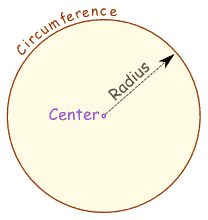A circle is easy to make: Draw a curve that is "radius" away  from a central point. And so: All points are the same distance from the center.

## You Can Draw It Yourself

Put a pin in a board, put a loop of string around it, and insert a pencil into the loop. Keep the string stretched and draw the circle!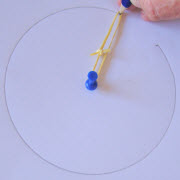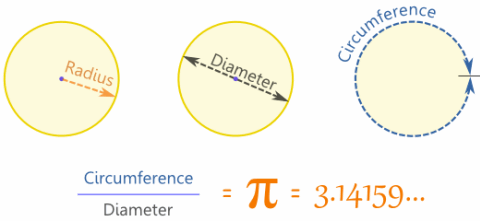The Radius is the distance from the center to the edge.

The Diameter starts at one side of the circle, goes through the center and ends on the other side.

The Circumference is the distance around the edge of the circle.

And here is the really cool thing:

When you divide the circumference by the diameter you get 3,141592654...
which is the number π (Pi)

 So when the diameter is 1, the circumference is 3,141592654...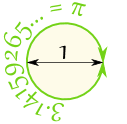We can say:

Circumference = π × Diameter

### Example: You walk around a circle which has a diameter of 100m, how far have you walked?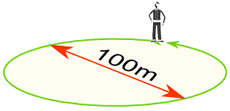Distance walked = Circumference = π × 100m = 314m (to the nearest m)

Also note that the Diameter is twice the Radius:

And so this is also true:

Circumference = 2 × π × Radius

## Remembering

• Radius is the shortest word
• Diameter is longer (and is 2 × Radius)
• Circumference is the longest (and is π × Diameter)

## Definition

 The circle is a plane shape (two dimensional): And the definition of a circle is: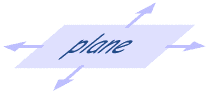The set of all points on a plane that are a fixed distance from a center.

## Area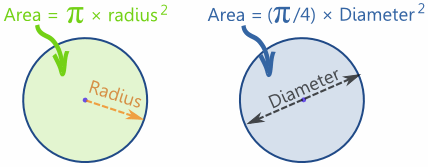The area of a circle is π times the radius squared, which is written:

A = π r2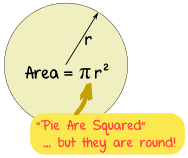(even though pies are usually round)

Or, in relation to Diameter:

A = (π/4) × D2

### Example: What is the area of a circle with radius of 1,2 m ?

A = π × r2

A = π × 1,22

A = π × (1,2 × 1,2)

A = 3,14159... × 1,44 = 4,52 (to 2 decimals)

### Area Compared to a Square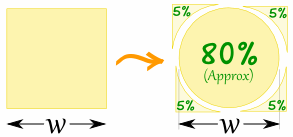A circle has about 80% of the area of a similar-width square.
The actual value is (π/4) = 0,785398... = 78,5398...%

## Names

Because people have studied circles for thousands of years special names have come about.

Nobody wants to say "that line that starts at one side of the circle, goes through the center and ends on the other side" when a word like "Diameter" would do.

So here are the most common special names: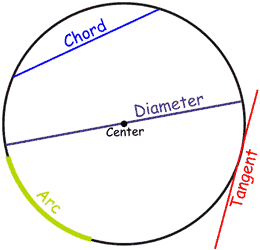## Lines

A line that goes from one point to another on the circle's circumference is called a Chord.

If that line passes through the center it is called aDiameter.

A line that "just touches" the circle as it passes by is called a Tangent.

And a part of the circumference is called an Arc.

## Slices

There are two main "slices" of a circle

The "pizza" slice is called a Sector.

And the slice made by a chord is called a Segment.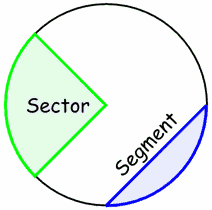## Common Sectors

The Quadrant and Semicircle are two special types of Sector: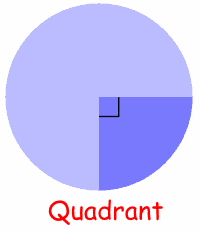Quarter of a circle is called a Quadrant. Half a circle is called a Semicircle.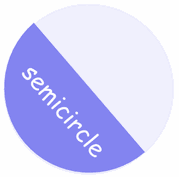## Inside and Outside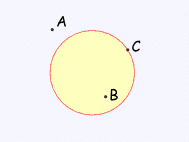A circle has an inside and an outside (of course!). But it also has an "on", because you could be right on the circle. Example: "A" is outside the circle, "B" is inside the circle and "C" is on the circle.